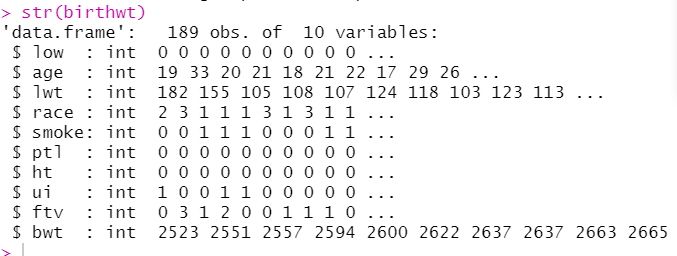V1

2023/03/17阅读：17主题：橙心

# R 医学统计学--T 检验

### 基本概念

T 检验，又称为学生 t 检验(Student's t-test)，主要适用于样本含量较小（例如 n < 30），总体标准差 σ 未知的正态分布。

### 主要应用

1. 单样本检验：检验一个正态分布的总体的均值是否在满足零假设的值之内，例如检验一群军校男生的身高的平均是否符合全国标准的 170 公分界线。
2. 独立样本 t 检验（双样本）：其零假设为两个正态分布的总体的均值之差为某实数，例如检验二群人之平均身高是否相等。若两总体的方差是相等的情况下（同质方差），自由度为两样本数相加再减二；若为异方差（总体方差不相等），自由度则为 Welch 自由度，此情况下有时被称为 Welch 检验。
3. 配对样本 t 检验（成对样本 t 检验）：检验自同一总体抽出的成对样本间差异是否为零。例如，检测一位病人接受治疗前和治疗后的肿瘤尺寸大小。若治疗是有效的，我们可以推定多数病人接受治疗后，肿瘤尺寸将缩小。
4. 检验一回归模型的偏回归系数是否显著不为零，即检验解释变量 X 是否存在对被解释变量 Y 的解释能力，其检验统计量称之为 t-比例（t-ratio）

## 单样本 T 检验

#### 满足条件

1. 观测变量为连续变量；
2. 观测值相互独立；
3. 观测变量接近正态分布。

``##T检验library(dplyr)library(MASS)str(birthwt)##查看数据结构``有 189 个观察单位，10 个变量，其中 bwt 为新生儿出生体重，也是我们要检验的变量，bwt 满足连续性数值，数据独立的条件。

``> ##检验数据是否符合正态分布> shapiro.test(birthwt\$bwt) Shapiro-Wilk normality testdata:  birthwt\$bwtW = 0.99244, p-value = 0.4353``

P>0.05，数据满足正态分布的要求

``> #独立单样本t检验> t.test(birthwt\$bwt,mu=3000)#已知总体均数为3000的单样本t检验 One Sample t-testdata:  birthwt\$bwtt = -1.0447, df = 188, p-value = 0.2975alternative hypothesis: true mean is not equal to 300095 percent confidence interval: 2839.952 3049.222sample estimates:mean of x 2944.587``

## 两独立样本 T 检验

#### 满足条件

1. 数据为连续型数据；
2. 各组数据均为正态分布；
3. 各组数据满足方差齐性；
4. 观测数据满足独立性。

``> #独立两样本t检验> ##检验两样本正态性> group1 <- birthwt\$bwt[birthwt\$smoke==0]#未吸烟组命名为group1> group2 <- birthwt\$bwt[birthwt\$smoke==1]#吸烟组命名为group2> shapiro.test(group1) Shapiro-Wilk normality testdata:  group1W = 0.98694, p-value = 0.3337> shapiro.test(group2) Shapiro-Wilk normality testdata:  group2W = 0.98296, p-value = 0.4195``

``> ##检验两样本方差齐性> var.test(bwt ~ smoke,data = birthwt) F test to compare two variancesdata:  bwt by smokeF = 1.3019, num df = 114, denom df = 73, p-value = 0.2254alternative hypothesis: true ratio of variances is not equal to 195 percent confidence interval: 0.8486407 1.9589574sample estimates:ratio of variances          1.301927``

``> t.test(bwt ~ smoke,var.equal=T,data = birthwt)#数据不满足方差齐性时——var.equal = F Two Sample t-testdata:  bwt by smoket = 2.6529, df = 187, p-value = 0.008667alternative hypothesis: true difference in means between group 0 and group 1 is not equal to 095 percent confidence interval:  72.75612 494.79735sample estimates:mean in group 0 mean in group 1       3055.696        2771.919``

## 配对样本 T 检验

#### 满足条件

1. 观测变量为连续变量。
2. 分组变量包含两个分类、且相关（配对）。
3. 两个相关（配对）组别间观测变量的差值近似服从正态分布。

``> DLD<- readxl::read_xlsx( "TDLD.xlsx" )> DLD# A tibble: 20 x 2    LDL1  LDL2   <dbl> <dbl> 1  6.05  4.75 2  5.12  3.25 3  7.03  5.15 4  6.18  4.51 5  5.19  3.69 6  5.26  3.85 7  5.1   3.48 8  5.2   4.02 9  7.08  5.3610  6.11  4.9111  5.17  3.7612  5.24  3.3713  7.02  5.2514  5.11  4.0315  6.14  4.2816  5.17  3.7917  5.14  3.918  6.22  4.6519  5.15  3.520  5.21  4.16``

``> ##检验两配对样本差值是否满足正态性检验> shapiro.test(DLD\$LDL1-DLD\$LDL2) Shapiro-Wilk normality testdata:  DLD\$LDL1 - DLD\$LDL2W = 0.93472, p-value = 0.1902``

P=0.190>0.05，表明两配对样本差值满足正态分布

``> ##第一种方法> t.test(DLD\$LDL1-DLD\$LDL2,mu=0) One Sample t-testdata:  DLD\$LDL1 - DLD\$LDL2t = 24.497, df = 19, p-value = 7.75e-16alternative hypothesis: true mean is not equal to 095 percent confidence interval: 1.382359 1.640641sample estimates:mean of x   1.5115> ##第二种方法> t.test(DLD\$LDL1,DLD\$LDL2,paired = T) Paired t-testdata:  DLD\$LDL1 and DLD\$LDL2t = 24.497, df = 19, p-value = 7.75e-16alternative hypothesis: true difference in means is not equal to 095 percent confidence interval: 1.382359 1.640641sample estimates:mean of the differences                 1.5115``

### 参考资料



《医学统计学》[第五版]: 孙振球，徐勇勇，人民卫生出版社V1